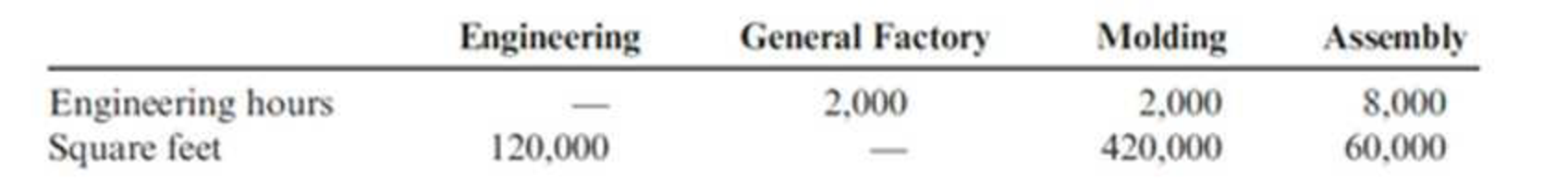# Macalister Corporation is developing departmental overhead rates based on direct labor hours for its two production departments—Molding and Assembly. The Molding Department employs 20 people, and the Assembly Department employs 80 people. Each person in these two departments works 2,000 hours per year. The production-related overhead costs for the Molding Department are budgeted at $190,000, and the Assembly Department costs are budgeted at$80,000. Two support departments—Engineering and General Factory—directly support the two production departments and have budgeted costs of $216,000 and$370,000, respectively. The production departments’ overhead rates cannot be determined until the support departments’ costs are properly allocated. The following schedule reflects the use of the Engineering Department’s and General Factory Department’s output by the various departments. For all requirements, round allocation ratios to four significant digits and round allocated costs to the nearest dollar. Required: 1. Calculate the overhead rates per direct labor hour for the Molding Department and the Assembly Department using the direct allocation method to charge the production departments for support department costs. 2. Calculate the overhead rates per direct labor hour for the Molding Department and the Assembly Department using the reciprocal method to charge support department costs to each other and to the production departments. 3. Explain the difference between the methods, and indicate the arguments generally presented to support the reciprocal method over the direct allocation method. (CMA adapted)### Cornerstones of Cost Management (C...

4th Edition
Don R. Hansen + 1 other
Publisher: Cengage Learning
ISBN: 9781305970663

#### Solutions

Chapter
Section### Cornerstones of Cost Management (C...

4th Edition
Don R. Hansen + 1 other
Publisher: Cengage Learning
ISBN: 9781305970663
Chapter 7, Problem 36P
Textbook Problem
698 views

## Macalister Corporation is developing departmental overhead rates based on direct labor hours for its two production departments—Molding and Assembly. The Molding Department employs 20 people, and the Assembly Department employs 80 people. Each person in these two departments works 2,000 hours per year. The production-related overhead costs for the Molding Department are budgeted at $190,000, and the Assembly Department costs are budgeted at$80,000. Two support departments—Engineering and General Factory—directly support the two production departments and have budgeted costs of $216,000 and$370,000, respectively. The production departments’ overhead rates cannot be determined until the support departments’ costs are properly allocated. The following schedule reflects the use of the Engineering Department’s and General Factory Department’s output by the various departments.For all requirements, round allocation ratios to four significant digits and round allocated costs to the nearest dollar.Required: 1. Calculate the overhead rates per direct labor hour for the Molding Department and the Assembly Department using the direct allocation method to charge the production departments for support department costs. 2. Calculate the overhead rates per direct labor hour for the Molding Department and the Assembly Department using the reciprocal method to charge support department costs to each other and to the production departments. 3. Explain the difference between the methods, and indicate the arguments generally presented to support the reciprocal method over the direct allocation method. (CMA adapted)

1.

To determine

Calculate the overhead rates per direct labor hour for the molding department and the assembly department using the direct allocation method.

### Explanation of Solution

Direct method of allocation: In direct method of allocation, companies assign support department costs only to the producing departments. This method is the simpler and straightforward for assigning support department costs.

 Engineering General factory Molding Assembly Department costs $216,000$370,000 $190,000$80,000 Allocation of: Engineering ($216,000)$0 (5)$43,200 (5)$172,800 General factory $0 ($370,000) (5)$323,750 (5)$46,250 Total overhead cost (a) $0$0 $556,950$299,050 Direct labor hours (b) 40,000 160,000 Overhead rate per DLH (ab) $14$2

Table (1)

Working notes:

(1)Calculate the allocation ratio of engineering hours to molding:

Allocation of engineering hours to molding}=Allocation of engineering hours to molding(Allocation of engineering hours to molding+Allocation of engineering hours to assembly)=2,0002,000+8,000=2,00010,000=0.2

(2)Calculate the allocation ratio of engineering hours to assembly:

Allocation of engineering hours toassembly}=Allocation of engineering hours to assembly(Allocation of engineering hours to molding+Allocation of engineering hours to assembly)=8,0002,000+8,000=8,00010,000=0

2.

To determine

Calculate the overhead rates per direct labor hour for the molding department and the assembly department using the reciprocal method.

3.

To determine

Explain the difference between the methods, and specify the arguments generally presented to support the reciprocal method over the direct allocation method.

### Still sussing out bartleby?

Check out a sample textbook solution.

See a sample solution

#### The Solution to Your Study Problems

Bartleby provides explanations to thousands of textbook problems written by our experts, many with advanced degrees!

Get Started